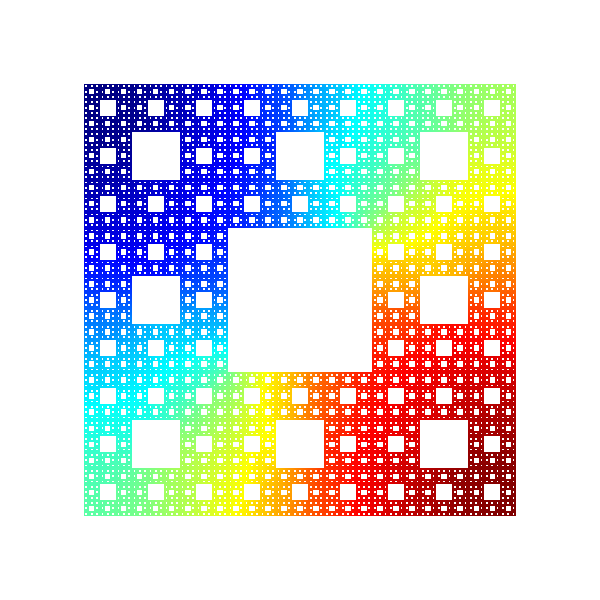# 6th Cornell Conference on Analysis, Probability, and Mathematical Physics on Fractals

June 13–17, 2017

## Abstracts of Courses

### A short course on analysis on Sierpinski Gasket type fractals

This course will describe the construction of a Laplacian on SG due to Kigami, the method of spectal decimation due to Fukushima and Shima, the generalization to other p.c.f. self-similar fractals, and as time allows some recent related results.

### Quantum symmetry breaking: scale anomaly and fractals

Scale invariance is a common property of our everyday environment. Its breaking gives rise to less common but beautiful structures like fractals. At the quantum level, breaking of continuous scale invariance is a remarkable exemple of quantum phase transition also known as scale anomaly. The general features of this transition will be presented at an elementary quantum mechanics level.

### Probability on fractals

This set of two lectures aims to cover the probabilistic aspect of analysis on fractals, complementing the tutorials by Bob Strichartz.

Using the language of Markov chains, I will explain the significance of harmonic functions, Green's functions, and heat kernels in the study of diffusions on fractals. I will also touch on various types of stopping times in Markov processes (hitting times, commute times, etc.) and their connections to electric network theory.

### Magnetic operators on fractals

We will build on the earlier courses to consider vector fields associated to a harmonic structure and then magnetic operators on pcf self similar fractals, concentrating on a few simple examples for which spectra of these operators can be determined by decimation methods. Results will be drawn from a number of sources but the presentation will emphasize connections to the Kigami approach to the energy and Laplacian.

### Resistance forms on fractal quantum graphs and related spaces

On classical self-similar fractals, such at the Sierpinski gasket and Sierpinski carpet, there is a successful theory of resistance forms and diffusion processes due to Kigami, Barlow, Bass, Hambly, Hino, Fukushima, Kususoka, Perkins et al. The construction of these forms and processes usually relies on some fixed point arguments. This talk will describe a method to construct symmetric local Dirichlet forms on some fractal spaces using simple algebras of functions that are in some sense 'inherited' from underlying Euclidean spaces. Example of such spaces include fractal quantum graphs, snowflake domains with interior and boundary diffusions, Laakso spaces, symmetric Barlow-Evans vermiculated spaces. The presentation will emphasize some simple common ideas that appear in joint research with Alonso-Ruiz, Hinz, Kelleher, Lancia, Steinhurst, Vernole.